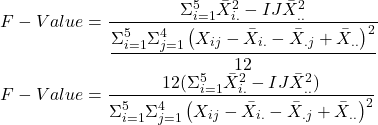## You have been given the task of designing a study concerning the lifetimes of five different types of electric motor. The initial question t

Question

You have been given the task of designing a study concerning the lifetimes of five different types of electric motor. The initial question to be addressed is whether there are differences in mean lifetime among the five types. There are 20 motors, four of each type, available for testing. A maximum of five motors can be tested in a day. The ambient temperature differs from day to day, and this can affect motor lifetime.
a. Describe how you would choose the five motors to test each day. Would you use a completely randomized design? Would you use any randomization at all?
b. If X;j represents the measured lifetime of a motor of type i tested on day j, express the test statistic for testing the null hypothesis of equal lifetimes in terms of the Xir

in progress 0
5 months 2021-08-25T18:25:40+00:00 1 Answers 0 views 0

a-As the only factor of interest is type of electric motor, thus instead of using 1-factor randomization, a randomized block design is used where at maximum one motor of each type is tested every day.

b- The test statistic is given as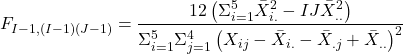Step-by-step explanation:

a-

This is done such that the days are considered as blocks and the randomization is only occuring within the block. The order of testing is random. However the condition is implemented such that one motor of each type is tested each day.

b-

The F-Value is given asHere MSA is given as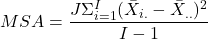Here

• I is the number of types which is 5
• J is the number of motors of each type which is 4
•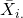is the row-wise mean which is given as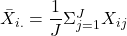•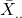is the sample grand mean which is given as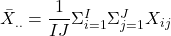Similarly MSAB is given as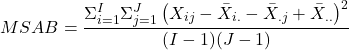Here

• I is the number of types which is 5
• J is the number of motors of each type which is 4
•is the row-wise mean which is given as•is the sample grand mean which is given as•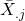is the column-wise mean which is given as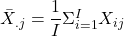•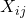is the any motor j of type i

By putting these values and simplifying, equation becomes: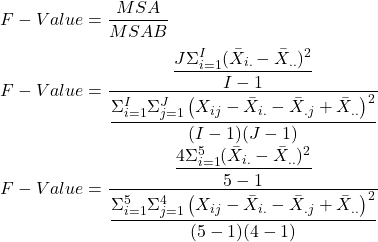This is further simplified as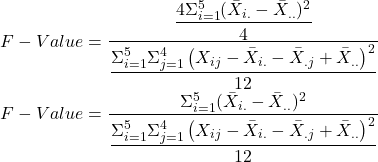The numerator can be written as